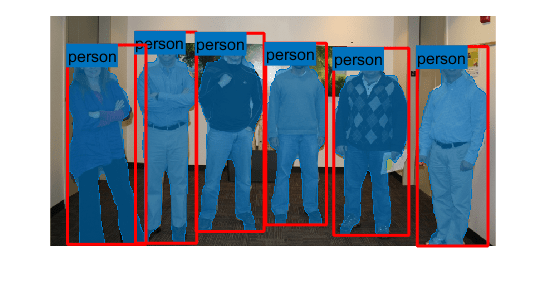segmentObjects

Segment objects using Mask R-CNN instance segmentation

Description

masks = segmentObjects(detector,I) detects object masks within a single image or an array of images, I, using a Mask R-CNN object detector.

masks = segmentObjects(detector,I,Name=Value) configures the segmentation using additional name-value arguments. For example, segmentObjects(detector,I,Threshold=0.9) specifies the detection threshold as 0.9.

[masks,labels] = segmentObjects(___) also returns the labels assigned to the detected objects.

[masks,labels,scores] = segmentObjects(___) also returns the detection score for each of the detected objects.

example

[masks,labels,scores,bboxes] = segmentObjects(___) also returns the location of segmented object as bounding boxes, bboxes.

Note

This function requires the Computer Vision Toolbox™ Model for Mask R-CNN Instance Segmentation. You can install the Computer Vision Toolbox Model for Mask R-CNN Instance Segmentation from Add-On Explorer. For more information about installing add-ons, see Get and Manage Add-Ons. To run this function, you will require the Deep Learning Toolbox™.

Examples

collapse all

detector =

ClassNames: {1×80 cell}
InputSize: [800 1200 3]
AnchorBoxes: [15×2 double]

Read a test image that includes objects that the network can detect, such as people.

Segment instances of objects using the Mask R-CNN object detector.

Overlay the detected object masks in blue on the test image. Display the bounding boxes in red and the object labels.

imshow(overlayedImage)
showShape("rectangle",boxes,Label=labels,LineColor=[1 0 0])Input Arguments

collapse all

Image or batch of images to segment, specified as one of these values.

Image TypeData Format
Single grayscale image2-D matrix of size H-by-W
Single color image3-D array of size H-by-W-by-3.
Batch of B grayscale or color images4-D array of size H-by-W-by-C-by-B. The number of color channels C is 1 for grayscale images and 3 for color images.

The height H and width W of each image must be greater than or equal to the input height h and width w of the network.

Name-Value Arguments

Specify optional pairs of arguments as Name1=Value1,...,NameN=ValueN, where Name is the argument name and Value is the corresponding value. Name-value arguments must appear after other arguments, but the order of the pairs does not matter.

Example: segmentObjects(detector,I,Threshold=0.9) specifies the detection threshold as 0.9.

Detection threshold, specified as a numeric scalar in the range [0, 1]. The Mask R-CNN object detector does not return detections with scores less than the threshold value. Increase this value to reduce false positives.

Maximum number of strongest region proposals, specified as a positive integer. Reduce this value to speed up processing time at the cost of detection accuracy. To use all region proposals, specify this value as Inf.

Select the strongest bounding box for each detected object, specified as a numeric or logical 1 (true) or 0 (false).

• true — Return the strongest bounding box per object. To select these boxes, the segmentObjects function calls the selectStrongestBboxMulticlass function, which uses nonmaximal suppression to eliminate overlapping bounding boxes based on their confidence scores.

• false — Return all detected bounding boxes. You can then create your own custom operation to eliminate overlapping bounding boxes.

Minimum size of a region containing an object, in pixels, specified as a two-element numeric vector of the form [height width]. By default, MinSize is the smallest object that the trained detector can detect. Specify this argument to reduce the computation time.

Maximum size of a region containing an object, in pixels, specified as a two-element numeric vector of the form [height width].

To reduce computation time, set this value to the known maximum region size for the objects being detected in the image. By default, MaxSize is set to the height and width of the input image, I.

Hardware resource for processing images with a network, specified as "auto", "gpu", or "cpu".

ExecutionEnvironmentDescription
"auto"Use a GPU if available. Otherwise, use the CPU. The use of GPU requires Parallel Computing Toolbox™ and a CUDA® enabled NVIDIA® GPU. For information about the supported compute capabilities, see GPU Support by Release (Parallel Computing Toolbox).
"gpu"Use the GPU. If a suitable GPU is not available, the function returns an error message.
"cpu"Use the CPU.

Output Arguments

collapse all

Objects masks, returned as a logical array of size H-by-W-by-M. H and W are the height and width of the input image I. M is the number of objects detected in the image. Each of the M channels contains the mask for a single detected object.

When I represents a batch of B images, masks is returned as a B-by-1 cell array. Each element in the cell array indicates the masks for the corresponding input image in the batch.

Objects labels, returned as an M-by-1 categorical vector where M is the number of detected objects in image I.

When I represents a batch of B images, then labels is a B-by-1 cell array. Each element is an M-by-1 categorical vector with the labels of the objects in the corresponding image.

Detection confidence scores, returned as an M-by-1 numeric vector, where M is the number of detected objects in image I. A higher score indicates higher confidence in the detection.

When I represents a batch of B images, then scores is a B-by-1 cell array. Each element is an M-by-1 numeric vector with the labels of the objects in the corresponding image.

Location of detected objects within the input image, returned as an M-by-4 matrix, where M is the number of detected objects in image I. Each row of bboxes contains a four-element vector of the form [x y width height]. This vector specifies the upper left corner and size of that corresponding bounding box in pixels.

When I represents a batch of B images, then bboxes is a B-by-1 cell array. Each element is an M-by-4 numeric matrix with the bounding boxes of the objects in the corresponding image.